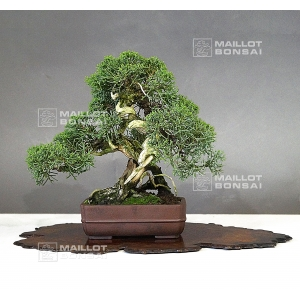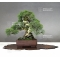Start your cart here complete your order on Maillot-Erable or Japon-Import and reduce your shipping costsThe Japanese Bonsai specialist
Direct order Contact Help / Services NewsletterJuniperus chinensis itoigawa 16050181

Juniperus chinensis itoigawaref. : 9915Product unavailable

Description

Height of the tree without its pot : 190 mm. Width of the branches : 255 mm. Non-enamelled pottery with a quality brown coloring of : 137x100x45 mm. Moyogi style. Juniper with a graceful silhouette, an exceptional shari on its twisted trunk and some jins. Itoigawa species with thin scales well canopy green colored, particularly vigorous and perfectly adapted to bonsai cultivation. Unlike the juniperus chinensis type, it loses only little foliage at the beginning of summer. Composed of a formed bark because this subject is aged of more than 40 years old. Not any strong wire or cutting injury. From Master Shiraichi’s nurseries. Exceptional subject! True bonsai with jin and native shari, do not confuse with the tanuki ones. Recently formed in February 2018 with copper wire by master Tomoya Nishikawa. Repoted in april 2017, you will have to do it again in april 2019, the same pot will be sufficient. Selected by Guy Maillot for its trunk’s quality and all the emotion given by this awesome tree. Admire the unique knotted trunk of this tree.

The whitening with jin liquid of the dead trunks softly colored with  charcoal will give a beautiful ash coloring.

Shelf non-included. These pictures were taken in May 2018.

#with 8.4 #bonsai 4.6 #juniperus 3.9 #chinensis 3.9 #itoigawa 3.8 #trunk 3.5 #this 3.4 #tree 3.4 #will 3.4 #exceptional 3.1

Formule
(( ROUND((CHAR_LENGTH(b.article_nom)-CHAR_LENGTH(REPLACE(b.article_nom, 'with', '')))/LENGTH('with')) + ROUND((CHAR_LENGTH(b.article_description)-CHAR_LENGTH(REPLACE(b.article_description, 'with', '')))/LENGTH('with')) ) * 8.4) + (( ROUND((CHAR_LENGTH(b.article_nom)-CHAR_LENGTH(REPLACE(b.article_nom, 'chinensis', '')))/LENGTH('chinensis')) + ROUND((CHAR_LENGTH(b.article_description)-CHAR_LENGTH(REPLACE(b.article_description, 'chinensis', '')))/LENGTH('chinensis')) ) * 3.9) + (( ROUND((CHAR_LENGTH(b.article_nom)-CHAR_LENGTH(REPLACE(b.article_nom, 'juniperus', '')))/LENGTH('juniperus')) + ROUND((CHAR_LENGTH(b.article_description)-CHAR_LENGTH(REPLACE(b.article_description, 'juniperus', '')))/LENGTH('juniperus')) ) * 3.9) + (( ROUND((CHAR_LENGTH(b.article_nom)-CHAR_LENGTH(REPLACE(b.article_nom, 'itoigawa', '')))/LENGTH('itoigawa')) + ROUND((CHAR_LENGTH(b.article_description)-CHAR_LENGTH(REPLACE(b.article_description, 'itoigawa', '')))/LENGTH('itoigawa')) ) * 3.8) + (( ROUND((CHAR_LENGTH(b.article_nom)-CHAR_LENGTH(REPLACE(b.article_nom, 'trunk', '')))/LENGTH('trunk')) + ROUND((CHAR_LENGTH(b.article_description)-CHAR_LENGTH(REPLACE(b.article_description, 'trunk', '')))/LENGTH('trunk')) ) * 3.5) + (( ROUND((CHAR_LENGTH(b.article_nom)-CHAR_LENGTH(REPLACE(b.article_nom, 'this', '')))/LENGTH('this')) + ROUND((CHAR_LENGTH(b.article_description)-CHAR_LENGTH(REPLACE(b.article_description, 'this', '')))/LENGTH('this')) ) * 3.4) + (( ROUND((CHAR_LENGTH(b.article_nom)-CHAR_LENGTH(REPLACE(b.article_nom, 'tree', '')))/LENGTH('tree')) + ROUND((CHAR_LENGTH(b.article_description)-CHAR_LENGTH(REPLACE(b.article_description, 'tree', '')))/LENGTH('tree')) ) * 3.4) + (( ROUND((CHAR_LENGTH(b.article_nom)-CHAR_LENGTH(REPLACE(b.article_nom, 'will', '')))/LENGTH('will')) + ROUND((CHAR_LENGTH(b.article_description)-CHAR_LENGTH(REPLACE(b.article_description, 'will', '')))/LENGTH('will')) ) * 3.4) + (( ROUND((CHAR_LENGTH(b.article_nom)-CHAR_LENGTH(REPLACE(b.article_nom, 'exceptional', '')))/LENGTH('exceptional')) + ROUND((CHAR_LENGTH(b.article_description)-CHAR_LENGTH(REPLACE(b.article_description, 'exceptional', '')))/LENGTH('exceptional')) ) * 3.1) + (( ROUND((CHAR_LENGTH(b.article_nom)-CHAR_LENGTH(REPLACE(b.article_nom, 'coloring', '')))/LENGTH('coloring')) + ROUND((CHAR_LENGTH(b.article_description)-CHAR_LENGTH(REPLACE(b.article_description, 'coloring', '')))/LENGTH('coloring')) ) * 2.8)

Secure paymentDelivery

Our logistic partners :